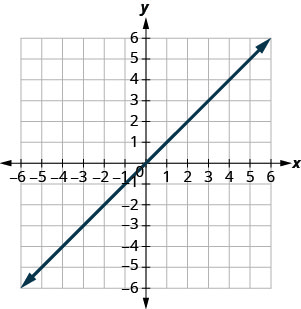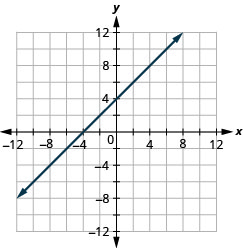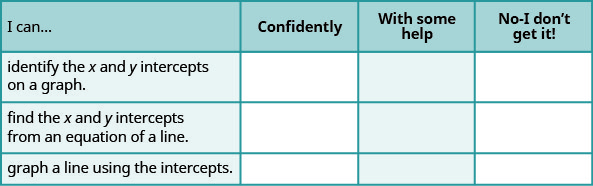$$\newcommand{\id}{\mathrm{id}}$$ $$\newcommand{\Span}{\mathrm{span}}$$ $$\newcommand{\kernel}{\mathrm{null}\,}$$ $$\newcommand{\range}{\mathrm{range}\,}$$ $$\newcommand{\RealPart}{\mathrm{Re}}$$ $$\newcommand{\ImaginaryPart}{\mathrm{Im}}$$ $$\newcommand{\Argument}{\mathrm{Arg}}$$ $$\newcommand{\norm}{\| #1 \|}$$ $$\newcommand{\inner}{\langle #1, #2 \rangle}$$ $$\newcommand{\Span}{\mathrm{span}}$$

# 4.3E: Exercises

•• OpenStax
• OpenStax
$$\newcommand{\vecs}{\overset { \rightharpoonup} {\mathbf{#1}} }$$ $$\newcommand{\vecd}{\overset{-\!-\!\rightharpoonup}{\vphantom{a}\smash {#1}}}$$$$\newcommand{\id}{\mathrm{id}}$$ $$\newcommand{\Span}{\mathrm{span}}$$ $$\newcommand{\kernel}{\mathrm{null}\,}$$ $$\newcommand{\range}{\mathrm{range}\,}$$ $$\newcommand{\RealPart}{\mathrm{Re}}$$ $$\newcommand{\ImaginaryPart}{\mathrm{Im}}$$ $$\newcommand{\Argument}{\mathrm{Arg}}$$ $$\newcommand{\norm}{\| #1 \|}$$ $$\newcommand{\inner}{\langle #1, #2 \rangle}$$ $$\newcommand{\Span}{\mathrm{span}}$$ $$\newcommand{\id}{\mathrm{id}}$$ $$\newcommand{\Span}{\mathrm{span}}$$ $$\newcommand{\kernel}{\mathrm{null}\,}$$ $$\newcommand{\range}{\mathrm{range}\,}$$ $$\newcommand{\RealPart}{\mathrm{Re}}$$ $$\newcommand{\ImaginaryPart}{\mathrm{Im}}$$ $$\newcommand{\Argument}{\mathrm{Arg}}$$ $$\newcommand{\norm}{\| #1 \|}$$ $$\newcommand{\inner}{\langle #1, #2 \rangle}$$ $$\newcommand{\Span}{\mathrm{span}}$$

## Practice Makes Perfect

Identify the x- and y- Intercepts on a Graph

In the following exercises, find the x- and y- intercepts on each graph.

##### Exercise $$\PageIndex{1}$$(3,0),(0,3)

##### Exercise $$\PageIndex{2}$$##### Exercise $$\PageIndex{3}$$(5,0),(0,−5)

##### Exercise $$\PageIndex{4}$$##### Exercise $$\PageIndex{5}$$(−2,0),(0,−2)

##### Exercise $$\PageIndex{6}$$##### Exercise $$\PageIndex{7}$$(−1,0),(0,1)

##### Exercise $$\PageIndex{8}$$##### Exercise $$\PageIndex{9}$$(6,0),(0,3)

##### Exercise $$\PageIndex{10}$$##### Exercise $$\PageIndex{11}$$(0,0)

##### Exercise $$\PageIndex{12}$$Find the x- and y- Intercepts from an Equation of a Line

In the following exercises, find the intercepts for each equation.

x+y=4

(4,0),(0,4)

x+y=3

x+y=−2

(−2,0),(0,−2)

x+y=−5

x–y=5

(5,0),(0,−5)

x–y=1

x–y=−3

(−3,0),(0,3)

x–y=−4

x+2y=8

(8,0),(0,4)

x+2y=10

3x+y=6

(2,0),(0,6)

3x+y=9

x–3y=12

(12,0),(0,−4)

x–2y=8

4x–y=8

(2,0),(0,−8)

5x–y=5

2x+5y=10

(5,0),(0,2)

2x+3y=6

3x–2y=12

(4,0),(0,−6)

3x–5y=30

##### Exercise $$\PageIndex{33}$$

$$y=\frac{1}{3} x+1$$

(-3,0),(0,1)

##### Exercise $$\PageIndex{34}$$

$$y=\frac{1}{4} x-1$$

##### Exercise $$\PageIndex{35}$$

$$y=\frac{1}{5} x+2$$

(−10,0),(0,2)

##### Exercise $$\PageIndex{36}$$

$$y=\frac{1}{3} x+4$$

y=3x

(0,0)

y=-2x

y=-4x

(0,0)

##### Exercise $$\PageIndex{40}$$

y=5x

Graph a Line Using the Intercepts

In the following exercises, graph using the intercepts.

##### Exercise $$\PageIndex{41}$$

$$-x+5 y=10$$##### Exercise $$\PageIndex{42}$$

$$-x+4 y=8$$

##### Exercise $$\PageIndex{43}$$

$$x+2 y=4$$##### Exercise $$\PageIndex{44}$$

$$x+2 y=6$$

##### Exercise $$\PageIndex{45}$$

$$x+y=2$$##### Exercise $$\PageIndex{46}$$

$$x+y=5$$

##### Exercise $$\PageIndex{47}$$

$$x+y=-3$$##### Exercise $$\PageIndex{48}$$

$$x+y=-1$$

##### Exercise $$\PageIndex{49}$$

$$x-y=1$$##### Exercise $$\PageIndex{50}$$

$$x-y=2$$

##### Exercise $$\PageIndex{51}$$

$$x-y=-4$$##### Exercise $$\PageIndex{52}$$

$$x-y=-3$$

##### Exercise $$\PageIndex{53}$$

$$4 x+y=4$$##### Exercise $$\PageIndex{54}$$

$$3 x+y=3$$

##### Exercise $$\PageIndex{55}$$

$$2 x+4 y=12$$##### Exercise $$\PageIndex{56}$$

$$3 x+2 y=12$$

##### Exercise $$\PageIndex{57}$$

$$3 x-2 y=6$$##### Exercise $$\PageIndex{58}$$

$$5 x-2 y=10$$

##### Exercise $$\PageIndex{59}$$

$$2 x-5 y=-20$$##### Exercise $$\PageIndex{60}$$

$$3 x-4 y=-12$$

##### Exercise $$\PageIndex{61}$$

$$3 x-y=-6$$##### Exercise $$\PageIndex{62}$$

$$2 x-y=-8$$

##### Exercise $$\PageIndex{63}$$

$$y=-2 x$$##### Exercise $$\PageIndex{64}$$

$$y=-4 x$$

##### Exercise $$\PageIndex{65}$$

$$y=x$$y=3x

## Everyday Math

##### Exercise $$\PageIndex{67}$$

Road trip. Damien is driving from Chicago to Denver, a distance of 1000 miles. The x- axis on the graph below shows the time in hours since Damien left Chicago. The y- axis represents the distance he has left to drive.1. Find the x- and y- intercepts.
2. Explain what the x- and y- intercepts mean for Damien.
1. (0,1000),(15,0)
2. At (0,1000), he has been gone 0 hours and has 1000 miles left. At (15,0), he has been gone 15 hours and has 0 miles left to go.
##### Exercise $$\PageIndex{68}$$

Road trip. Ozzie filled up the gas tank of his truck and headed out on a road trip. The x- axis on the graph below shows the number of miles Ozzie drove since filling up. The y- axis represents the number of gallons of gas in the truck’s gas tank.1. Find the x- and y- intercepts.
2. Explain what the x- and y- intercepts mean for Ozzie.

## Writing Exercises

##### Exercise $$\PageIndex{69}$$

How do you find the $$x$$ -intercept of the graph of $$3 x-2 y=6 ?$$

##### Exercise $$\PageIndex{70}$$

Do you prefer to use the method of plotting points or the method using the intercepts to graph the equation 4x+y=−4? Why?

##### Exercise $$\PageIndex{71}$$

Do you prefer to use the method of plotting points or the method using the intercepts to graph the equation $$y=\frac{2}{3}x−2$$? Why?

##### Exercise $$\PageIndex{72}$$

Do you prefer to use the method of plotting points or the method using the intercepts to graph the equation y=6? Why?

## Self Check

ⓐ After completing the exercises, use this checklist to evaluate your mastery of the objectives of this section.ⓑ What does this checklist tell you about your mastery of this section? What steps will you take to improve?

4.3E: Exercises is shared under a not declared license and was authored, remixed, and/or curated by OpenStax.